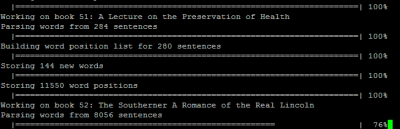## Handy Apply-based R Progress Bars

In a funny congruence with my post on R progress bars, Mark Heckmann just posted about some wrappers for the apply functions in R. He wrote up some functions that imitate `sapply`, `lapply` and `apply` but automatically add a progress bar so you can monitor the progress. They work very nice since you can just substitute his `apply_pb`‘s in place of R’s standard `apply`‘s. He says it’s a bit of a performance drag but after testing a bit it looks like they really shouldn’t add much overhead at all if there’s any major calculations inside the loop. Very handy.

## Progress Bars in R

Recently, I’ve had a lot of time consuming tasks running in R where it’s nice to know how the computer is doing. I usually just output the name of the current iteration or a dot or something but I finally decided I should figure out how to make a nice progress bar in R. It turns out it’s really simple since it’s already builtin with the `txtProgressBar` function. So you can do something like:

[R] numberSteps<-10 pb <- txtProgressBar(min = 0, max = numberSteps, style = 3) for(i in 1:numberSteps){ setTxtProgressBar(pb, i) Sys.sleep(1) } close(pb) [/R]That’s good enough for me but there’s also `winProgressBar` for a fancy Windows progress bar and `tkProgressBar` (in the `tcltk` package) if you really want to get fancy.

## Converting Between Gapped and NonGapped DNA Coordinates in R

I’ve been needing to convert coordinates in gapped DNA alignments back and forth to coordinates in the nongapped sequence a lot recently. For example, the T in AC–TGA–A is in the 5th position but in the gapless sequence ACTGAA it is 3rd. My first few tries (counting chars in regex’d substrings) at programming up a decent converters ended up not working very well with large datasets. Since I had to do it with 50,000 positions in a sequence with 2 million letters, I was running into a little trouble. But it ended up actually being a pretty easy problem in R so I thought I’d post it up in case anybody else is running into something similar. Also it’s a pretty good example why programming in R can be fun.

[R] gap2NoGap<-function(gapSeq,coords){ gapSeqSplit<-strsplit(gapSeq,'')[] nonDash<-gapSeqSplit!='-' newCoords<-cumsum(nonDash) return(newCoords[coords]) } [/R]

So the function takes a gapped sequence and the gapped coordinates. It splits the gapped sequence into an array of single characters and finds which ones are not -‘s. It then takes the cumulative sum at each element in the array (TRUE evaluates as 1 and FALSE as 0). This gives an array where each element gives the new gap-free coordinates. So the function just returns the appropriate new coordinate for each old coordinate.

[R] noGap2Gap<-function(gapSeq,coords){ gapSeqSplit<-strsplit(gapSeq,'')[] newCoords<-which(gapSeqSplit!='-') return(newCoords[coords]) } [/R]

This opposite conversion function again takes the gapped sequence and appropriately the nongapped coordinates. It splits the gapped sequence into an array of single characters and finds the indices of the characters which are not -‘s. Since we only stored the indices of nongap characters, this array gives the gapped coordinates for each nongap letter. So again the function can just returns the appropriate new coordinate for each old coordinate.

These ended up being pretty elegant in R (once I finally figured them out).

## How SINs (and Credit Card Numbers) Are Validated

A while back it was tax season in Canada and a friend of mine was trying to do his taxes online. But since he was foreign and didn’t have a Social Insurance Number (their equivalent of an SSN), the helpful webapp wouldn’t let him print the thing (of course it only informed him of that after he had already entered everything). We tried a few guesses before finally just using mine and crossing out my numbers after printing it. But I always wondered how the form knew our guesses were invalid. Luckily, I recently stumbled across a mention of how credit card numbers are validated.

It turns out SINs and credit card number are checked with something called the Luhn algorithm. Basically the algorithm just involves taking each digit, multiplying the second, fourth, sixth and so on digit from the right by 2 and adding up all the resulting digits (e.g. if 7 is multiplied by 2 then the resulting 14 is split into 1+4). If the sum of the digits is a multiple of 10, the number passes.

For example, to check 345678, you’d split it into 3,4,5,6,7,8. Then multiply 3, 5 and 7 by 2 to give 6, 4, 10, 6, 14, 8. Then split all the digits again to give 6, 4, 1, 0, 6, 1, 4, 8. That adds up to 30 so 345678 would be a valid credit card number (if there wasn’t a set length).

Just for fun, here’s a quick function in R to run the Luhn Algorithm on a number (or tell you the remainder so you can adjust):

[R] luhnCheck <- function(number,returnLogical=TRUE){ numbers <- gsub('[^0-9]','',as.character(number)) numbers <- as.numeric(strsplit(numbers,'')[]) selector<-seq(length(numbers)-1,1,-2) numbers[selector]<-numbers[selector]*2 numbers[numbers > 9] <- numbers[numbers > 9] – 9 remainder <- sum(numbers) %% 10 if(returnLogical) return(remainder==0) else return(remainder) } [/R]

So the next time some stupid web form needs a SIN number I’m going with 999999998.